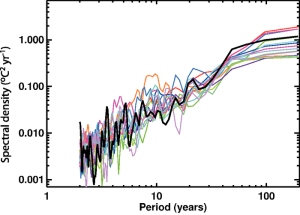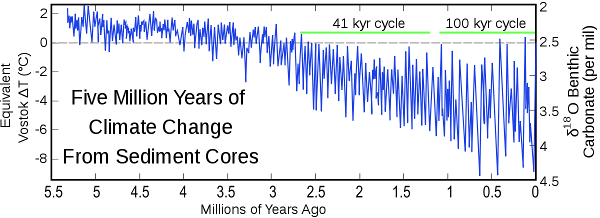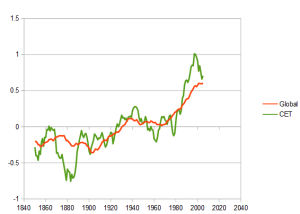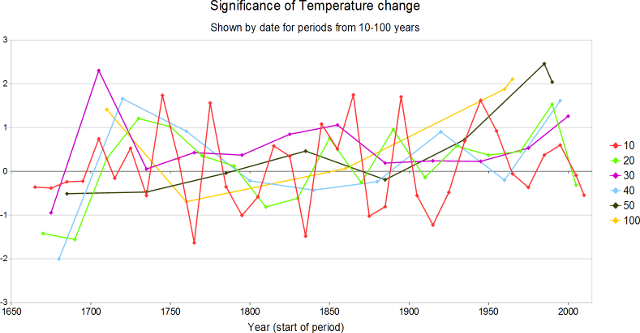# Proof: recent temperature trends are not abnormal

It has frequently been stated that 2oth century warming was “unprecedented” or “cannot be explained”. This article sets out to test this assertion on CET the longest available temperature series. I find the CET data rejects the hypothesis of ‘climate change’ (>58%) & current ‘global warming’ (>72%) and that overall global temperature has not changed significantly more than would be expected. I do however detect a marginally higher trend over a 50year period ending 2009 with about 2.5σ and a 35% chance of occurring normally within the dataset. However this is inconsistent with an established trend as progressively shorter periods toward the present time tend toward lower trends (40yr: 1.7σ, 30yr: 1.3σ, 20yr: 1.6σ, 10yr: -0.9σ).
I am therefore more than 58% certain that the data is consistent with natural variation and more than 73% certain that any current warming is within the normal range expected.

## Introduction

Many such as the IPCC have repeatedly asserted that we are seeing abnormal changes in the temperature particularly after the latter half of the 20th century (i.e. post the global cooling scare of the 1970s). In order to assess these statements I wanted to know the typical trends that we see in the climate record and to use these to make my own assessment of whether my assertion that “the temperature record is consistent with natural variation” can be supported from the data.
The reason I have not done this before, is that it is simply false to base any statistic on a simple standard deviation such as employed by Hansen who completely falsely came up with a figure that climate change was more than 99% likely to be caused by humans. To show how ridiculous this figure is, his statistic would also mean that the little ice, age, medieval warming and all the ice-ages are “human caused”.
The problem is that Hansen falsely used statistics that only work when the temperature of each year is totally unrelated to the previous years. And therefore any change common to a group of years would represent external forcing. However this is not how the real climate behaves. We have years, decades, centuries, even millennium with higher or lower temperatures. Therefore, in order to know what is “abnormal” or in the common language of climate alarmists what is “unprecedented” we must first know what is normal.
Only when we know what is normal can we have nay hope at all of spotting a departure from normality. So, when we only have global temperature data from around 1850 and only one full century to compare with itself, anyone who says “the twentieth century rise is abnormal” is either ignorant or being dishonest.

It is that simple.Variability of observed global mean temperature as a function of time-scale (°C2 yr–1)
from figure 9.7 IPCC (2007)

The problem as the IPCC know full well from their figure 9.7 of the 2007 report (shown right) is that the natural variation shown in global temperature varies dramatically depending on the period over when measurements are taken.
So, whilst it would be possible to make a valid statement about warming within any decade in the global temperature record, because we have a a sample of 16 decades to compare it with, it is nonsense to make any statement about century scale warming being “abnormal” when we only have one full century of data which must both serve to work out what is “normal”.
To illustrate how we must know “normality” let me take the extreme example of a 3C temperature change. Is this within the range of “normal variation”. The answer is that it all depends. Below is the estimated temperature change over an ice-age cycle.Here we can see that the suggested change of about 0.75C over the 20th century is completely insignificant compared to the 8C change that the globe experiences going through the ice-age cycle. So, a 3C change over 100,000 years would be entirely within the normal changes seen in the climate, but a 3C change seen in one decade would be extremely unusual in the available global instrumentation climate record.

## Aim of analysis

There is simply insufficient global temperature data available to test the hypothesis that 20th century warming is “abnormal”. However, temperature data is available regionally for longer periods which could test whether there has been any sign of unprecedented warming regionally. The longest of these is the Central England Temperature series which is available from about 1660.
I therefore decided to work out the typical trends over various periods and then used this to assess whether there were any “abnormal” trends in the record.
What makes my approach different is that I am not making any assumptions about how the climate behaves except that what is “normal” in any period should be “normal” within any similar length period. The key to this approach is that I am treating each time period as a different dataset. Therefore the appropriate test is to determine the variation typical in that time length and then to assess whether any period has a trend that is significantly outside the normal variation expected for that length of period.

##Does CET correlate with Global temperature

First however, I need to show that CET is a reasonal proxy for global temperature. To do this I will check how well CET is correlated global temperatures. A comparison of the two is shown right (offset to have zero average and with 11year moving average). Whilst the two have significant differences, the important trend referred to as “global warming” is present as well as the main 1970s “global cooling” dip which gave rise to the global cooling scare.
A calculation of correlation for the available data shows:

correlation coefficient R = 0.54

This indicates that moderate correlation is present. (And I will add this is much better than any tree-ring proxies).

## Method of Analysis

1. Data was the yearly average taken from Monthly_HadCET_mean.txt, 1659 to date
2. For each sample length (2, 5, 10, 20, 30, 40, 50, 100) the data was split into appropriate size samples starting from 1660.
3. Where insufficient data was available at the end it was either ignored or if close enough to avoid major overlap, the sample was taken starting at an earlier date. (See table below)
4. The slope was calculated for each sample.
5. The standard deviation of sample slopes were calculated for each sample length.
6. The standard deviation of all samples of each length was fitted to a polynomial against sample length. This produced a function estimating the expected standard deviation of slope for each sample length.
7. The slope from each sample was then normalised (to give unity standard deviation), so that when plotted the temperature trend in sample would be shown on a scale relative to the standard deviation of that sample length.

## Results

### Typical Trends

Below is the summary table of the typical variation of trend seen for each period/sample length in the CET dataset. The second row is figure derived from the model as detailed below. The last row is the typical change we would expect in a period of this length. So, for example we would normally see around 0.45C variation from century to century and 0.83 variation from decade to decade.

 Period (yrs) 2 5 10 20 30 40 50 100 1000 Variation (C/yr) 0.753 0.198 0.077 0.03 0.016 0.017 0.012 0.005 – Model (C/yr) 0.643 0.201 0.083 0.035 0.021 0.014 0.011 0.004 0.002 Model Period Change (C) 1.29 1 0.83 0.69 0.62 0.57 0.54 0.45 0.24

Variation versus sample length

Above is the variation given by the standard deviation of trend shown against the sample length (shown as period length). The figure on the left is shown with a log vertical scale so that all sample lengths can be seen. That to the right has a linear vertical scale showing the sample length of interest so that they can be compared with the model.
These figures shows that for the period lengths analysed there is a close fit to a polynomial with a general downward slope whose value is:

Standard Deviation (C/yr) = 1.55 × period -1.27

This close fit shows that no period significantly deviates from the modelled power law relationship. As a significant deviation would be expected if any period had seen significant change from natural this is the first evidence against the hypothesis of abnormal behaviour.

### Temperature Trend by year

In order to test the hypothesis of whether any abnormal trend exists, it was decided to first plot out the trends to see whether any abnormality was apparent. Below is a plot showing the normalised temperature trend for various lengths chunks by year.Note: Periods are plotted by average date

### Analysis

• 10 year (red) – shows no significant trend with peaks of around ±1.5σ being common. There is no indication that the rate of warming within any ten year period is increasing since 1950. Recent trend is smaller than expected and the last period is negative.
• 20 year (green) – has similar sized peaks up to ±1.5σ. The highest trend occurs at the end of the series but this is not dissimilar to the earlier peak around 1720 nor other length periods
• 30 year (mauve) – similar to changes shown in 20 year period length except earlier peak is one of the largest approaching 2.5σ. However the recent trend is much smaller than would be expected.
• 40 year (light blue) – again similar to 20 year period length
• 50 year (black) – if taken out of context this plot would appear to show a significant trend, but when seen in context the scale of change is not abnormal. This plot shows the largest trend of 2.46σ. Thus we would expect 90% of the 5 data points from five different periods (although not independent) to be less than this. Therefore this deviation whilst larger than others cannot be said to be significant.
• 100year (yellow) – is again similar to other plots

### Latest trend

 Measurement period (years) Trend relative to standard deviation End Year 10 -0.09 (-0.55) 2000-2009 (2005-2014) 20 1.53 (-0.32) 1990-2009 (1995-2014) 30 1.25 1985-2014 40 1975-2014 50 2.46 (2.03) 1960-2009 (1965-2014) 100 1.88 (2.01) 1910-2009 (1915-2914)

From the visual analysis it was apparent that the most likely sample period to represent abnormal behaviour was the 50 year period ending in 2009.
Calculation of abnormality
The question that needed to be determined was not whether any one period in series of measurement was high, but whether given so many different periods were assessed, whether we would expect to get one so high. This is because there are so many different periods over which a trend could be assessed. So if we find one period that happens to be high, if to find this result we had considered several hundred ways of calculating such a trend, then clearly there is a much higher chance than if there is one and only one figure.
To use the example, if the 50 year trend is high, but not the 40,30,20,10 year trends, is this significant?
Whilst a figure of 2.5 standard deviations means that such a large deviation is atypical (~99%), if we looked at thousands of periods we would expect to get around 10 or so periods exceeding the 2.5 standard deviations. So we need to know the number of different samples considered.
In total there were around 80 different periods considered. However obviously a 40 year period overlapping with a 50 year period is likely to be very similar. So, if we find a high trend in one we would expect it in the other. These sample are not independent.
So, how many discrete sample do I have present? I chose to estimate this using the principle of selected samples that are likely to be orthogonal. One such group of orthogonal sample can be constructed by doubling the period of length of each sample. This suggests periods of  2.5, 5, 10,20, 40, 80 etc. With hindsight I would repeat the analysis with such periods, however as I only need an estimate of the number of independent samples, I chose to count only the periods of 10, 20, 50 & 100 years as the “population of sample”. I also discarded samples from the end.
In total this gave an estimate of 61 unique samples and therefore the question became within these 61 samples how unusual was the highest value seen (the 50 year normalised trend ending 2009).
This was calculated as follows:

If P is the probability of any one sample being at the standard deviation
Probability all 61 samples are lower = P61

This was calculated for both “warming” as in “being so high” and also “climate change” as in “being either so high or so low” with the following resutls

 50 yr normalised trend ending Value (σ) Probabilty a sample lower Probabilty a sample closer to mean Probabilty all samples lower Probabilty all samples closer to mean 2009 2.46 99.3% 98.6% 65.0% 42.2% 2014 2.04 97.9% 95.8% 27.8%

## Discussion

The hypothesis I wish to test was whether there is any trends within the Central England Temperature series is inconsistent with natural variation.
Within this analysis one of 50 years ending in 2009 was sufficiently high to be considered as possibly showing abnormality. However when assessed statistically I found that the chance of this being abnormal climate variation was only 42.2%. Therefore the data does reject the null hypothesis which is that the dataset is due to natural variation.
However, if instead of “climate variation”, we only consider “warming”, there is a 65% probability that the single 50 year period ending in 2009 should be lower in a normal distribution. But, if the hypothesis of “current warming” is to be supported, it must not only be true in 2009 but also true in all later periods including the latest in 2014. Therefore the probability that the current 50 year trend (to 2014) is abnormal is only 27.8% which means that current warming is not supported from the CET dataset.
Given the correlation between CET and global temperatures, I can therefore conclude that it is unlikely that either current “global warming” or “climate change” is abnormal or departs significantly from what we expect of normal natural climatic variation.

This entry was posted in 1/f, Climate, My Best Articles and tagged , , , , . Bookmark the permalink.